# RD Sharma Solutions for Class 8 Maths Chapter 8 Division of Algebraic Expressions Exercise 8.5

In the previous exercise, we have seen that the long division method is quite lengthy and time-consuming. In this Exercise 8.5 of Chapter 8, we will discuss an alternative form of long division method. This form is concise and easy to use. The RD Sharma Solutions is prepared by our expert tutors at BYJU’S, which is one of the best reference material to prepare for exams and also helps students to strong command over the subject to score well in their exams. Students can download the pdf from the links given below.

## Download the pdf of RD Sharma Solutions for Class 8 Maths Exercise 8.5 Chapter 8 Division of Algebraic Expressions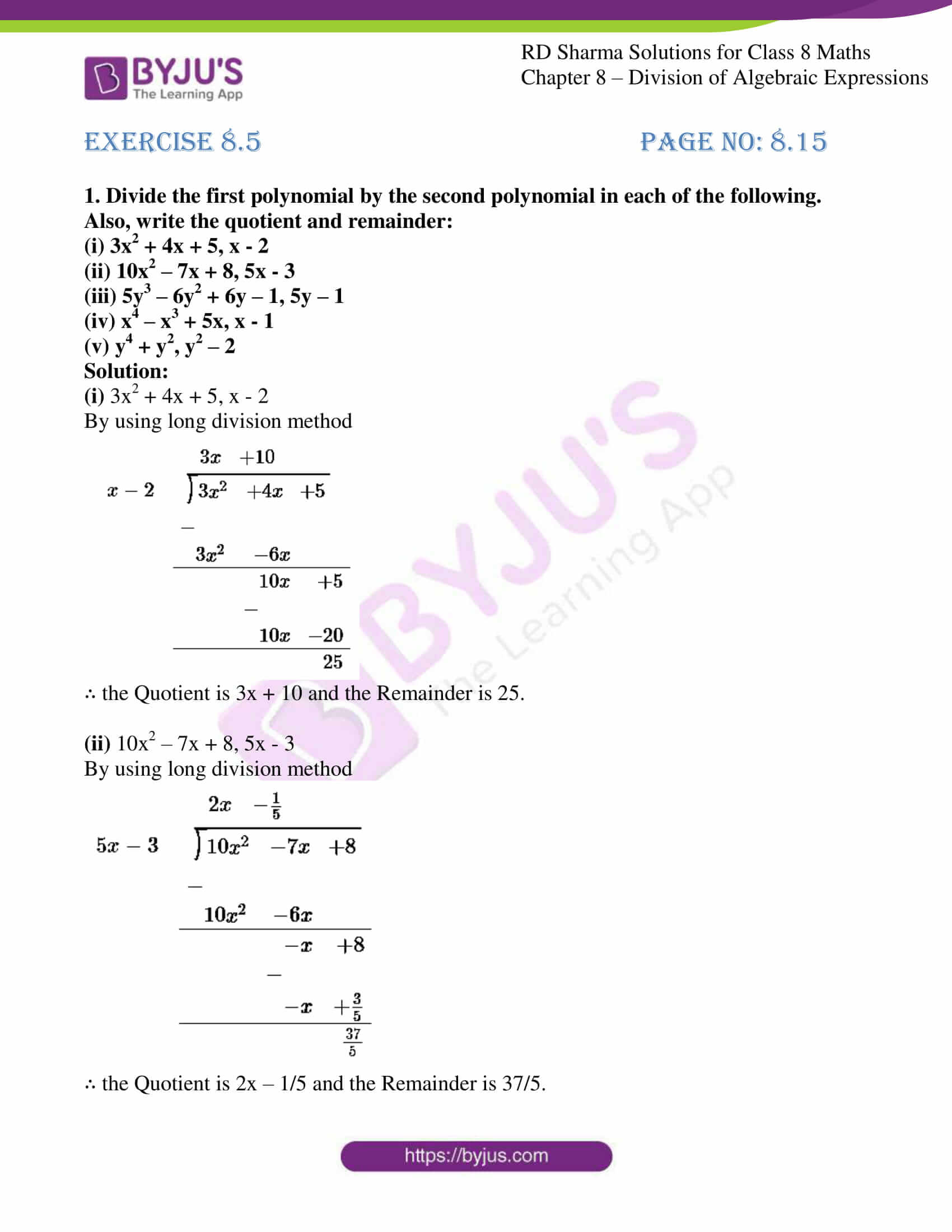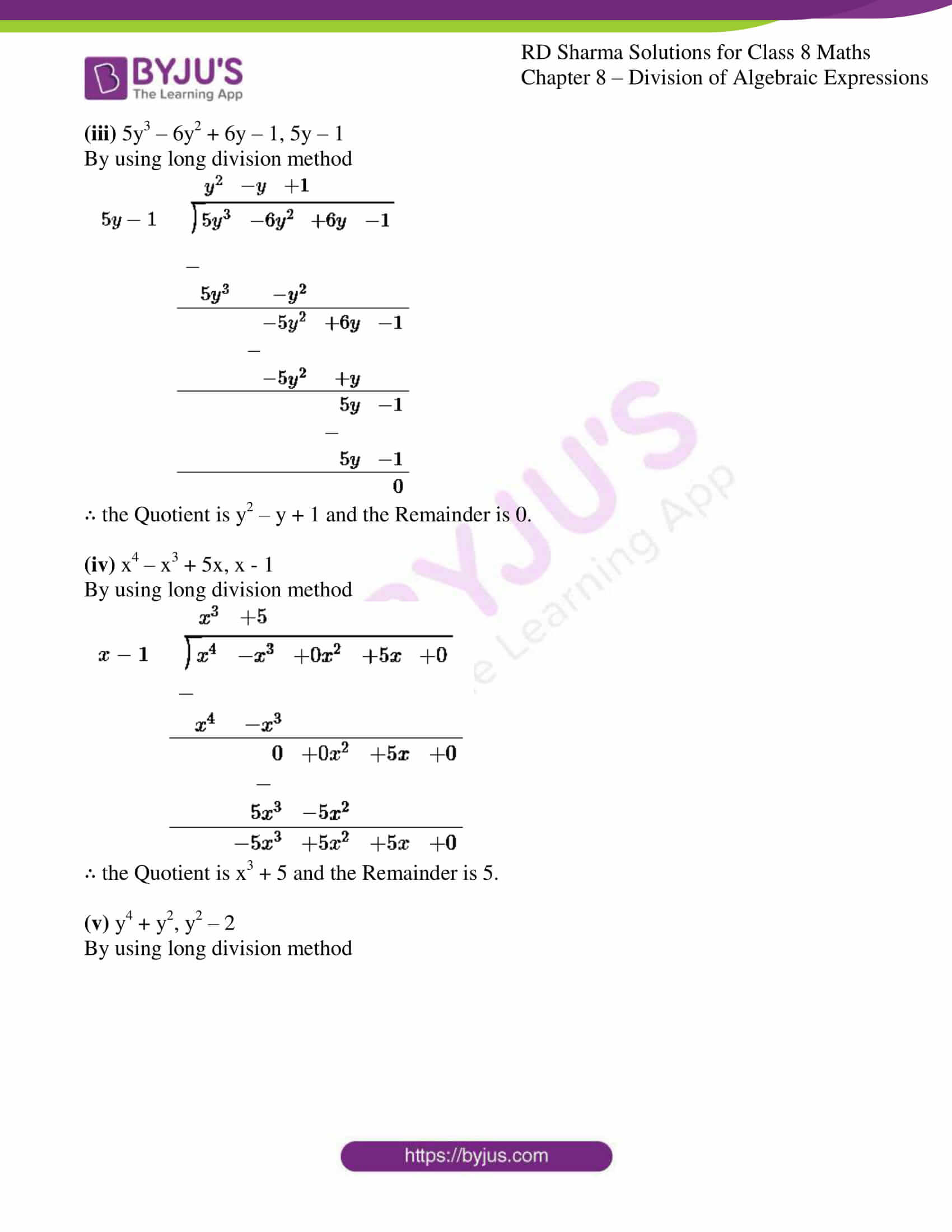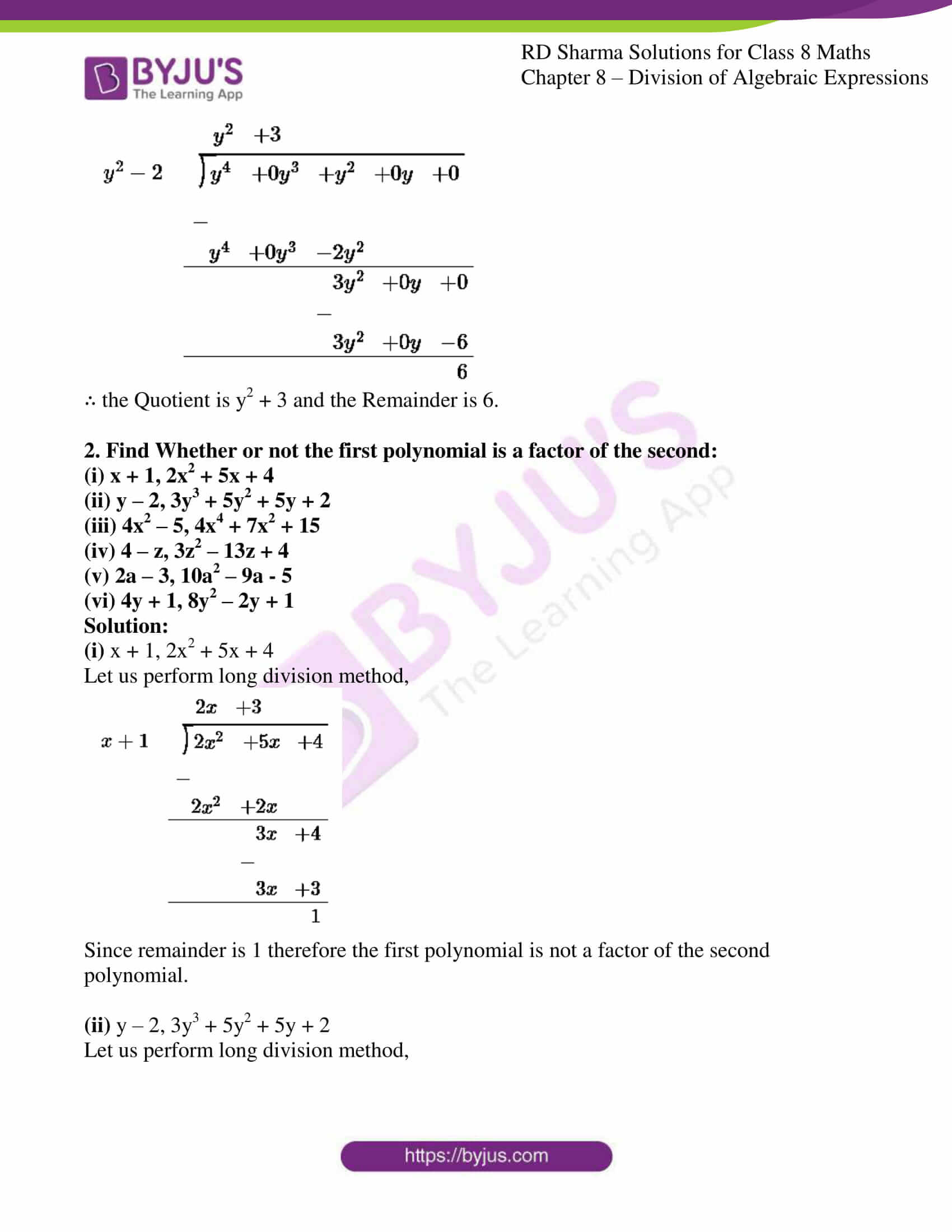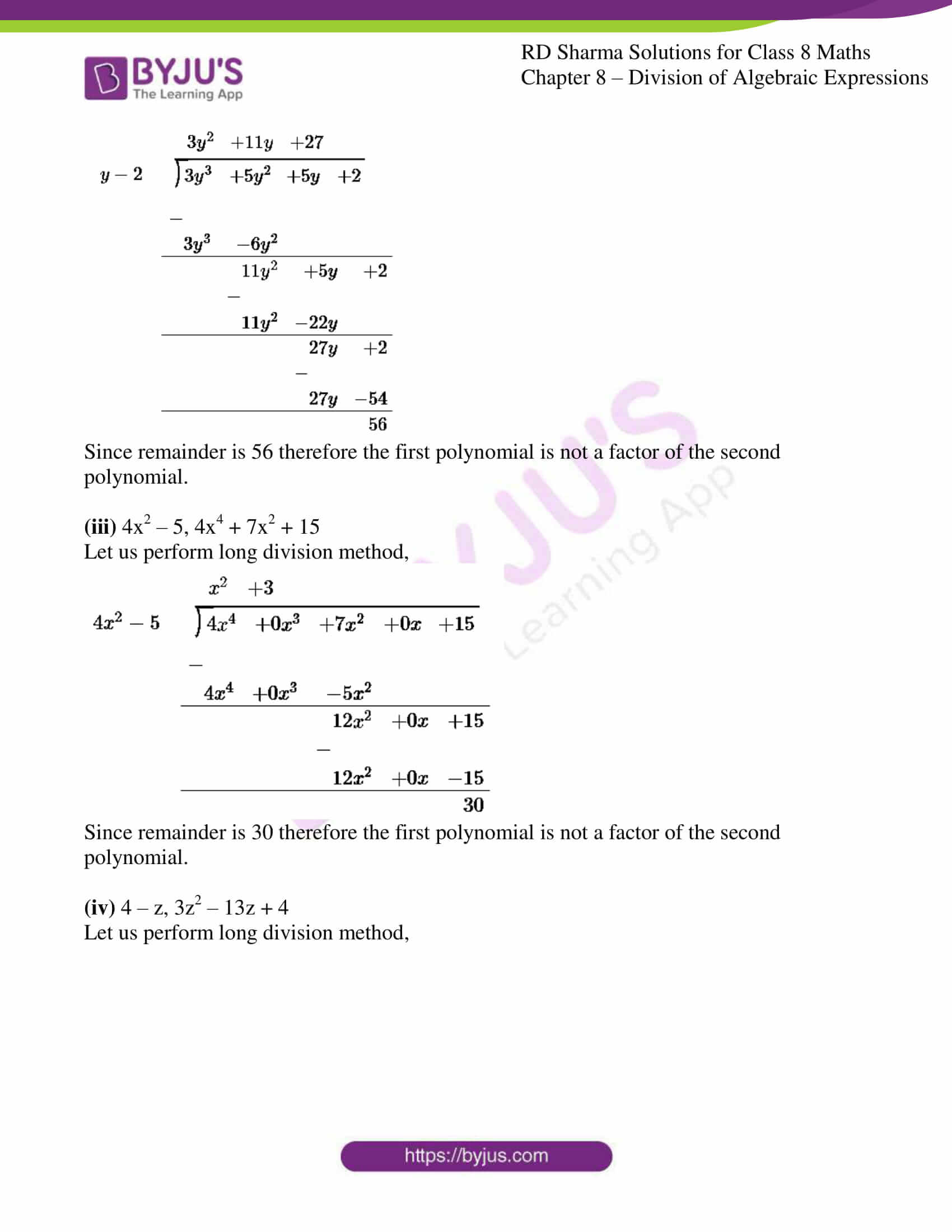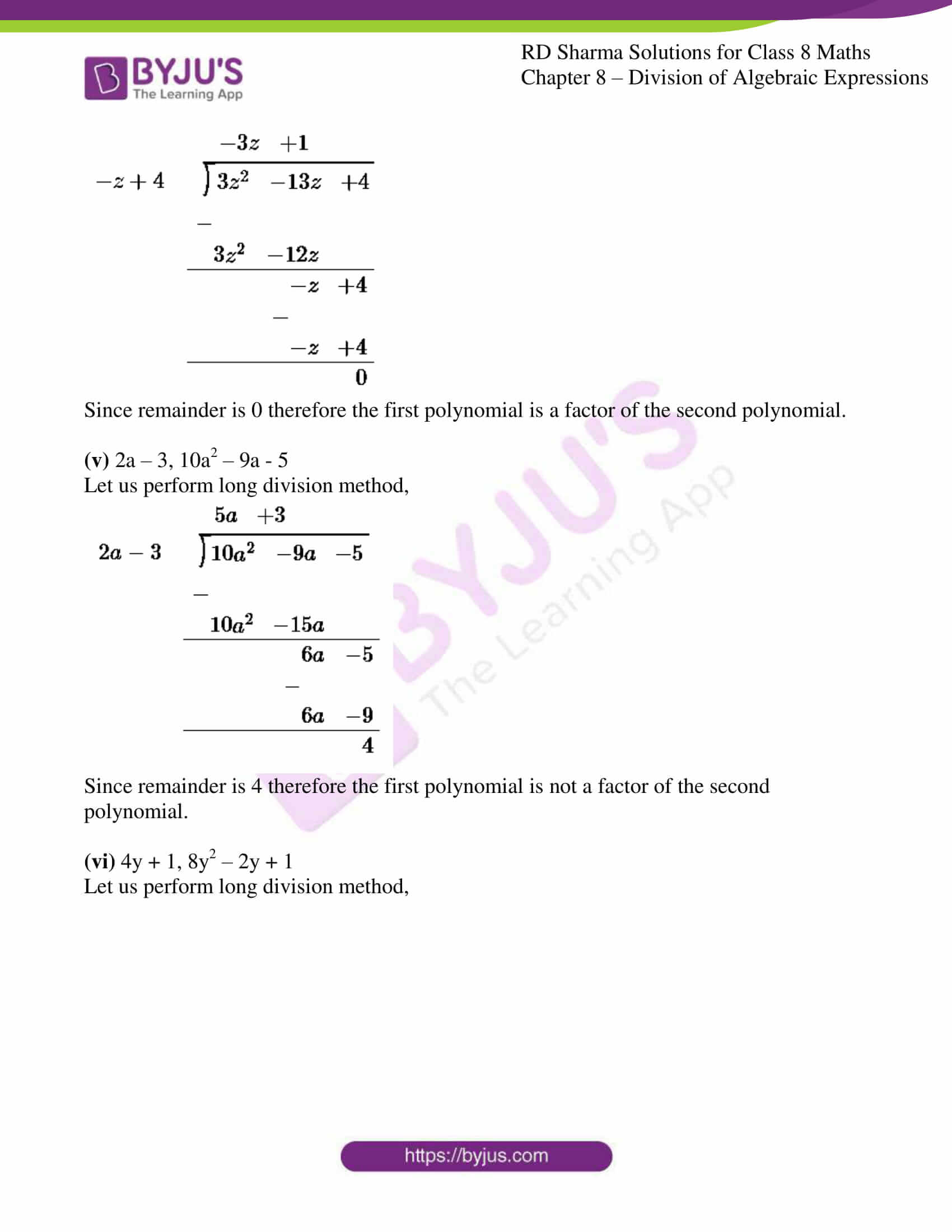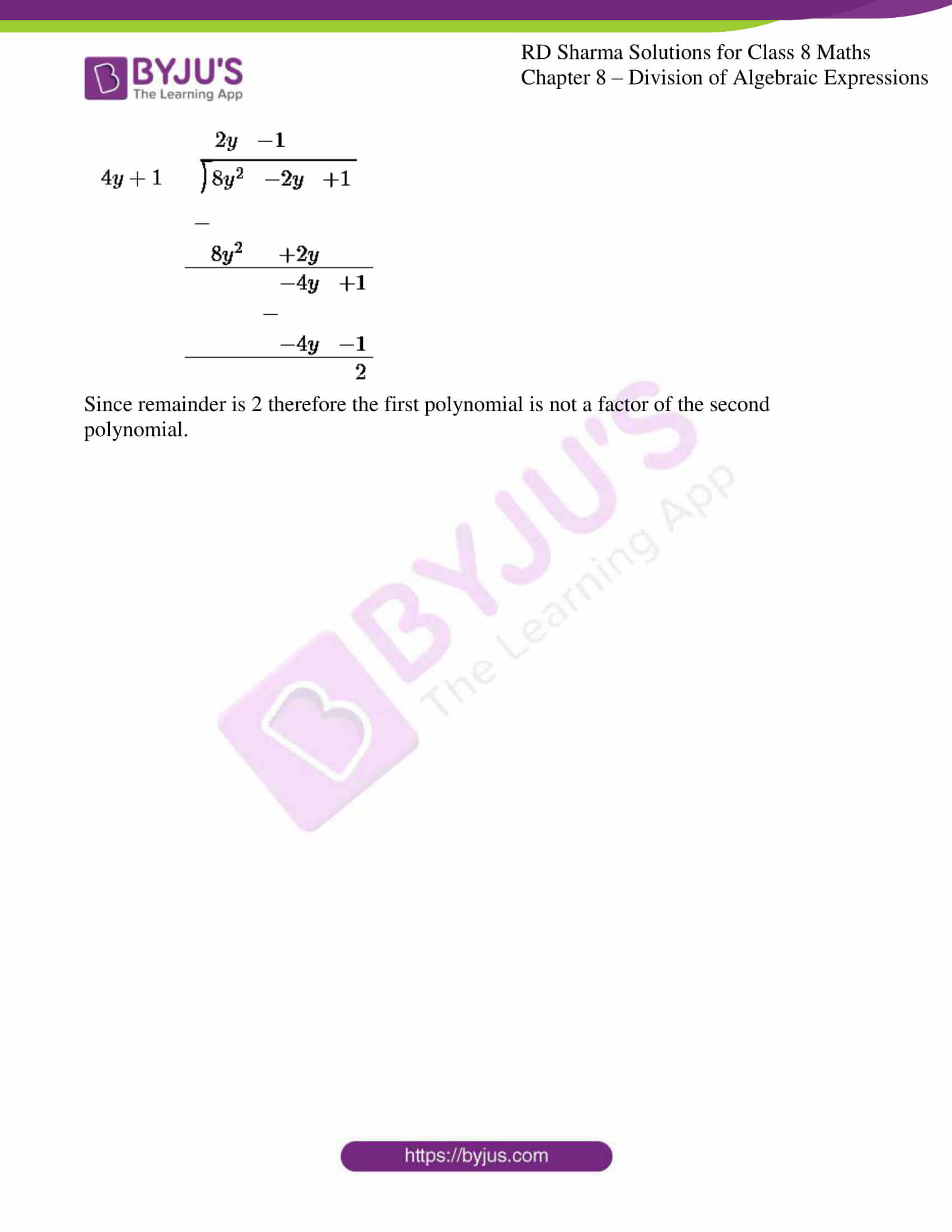### Access Answers to RD Sharma Solutions for Class 8 Maths Exercise 8.5 Chapter 8 Division of Algebraic Expressions

#### EXERCISE 8.5 PAGE NO: 8.15

1. Divide the first polynomial by the second polynomial in each of the following. Also, write the quotient and remainder:

(i) 3x2 + 4x + 5, x – 2

(ii) 10x2 – 7x + 8, 5x – 3

(iii) 5y3 – 6y2 + 6y – 1, 5y – 1

(iv) x4 – x3 + 5x, x – 1

(v) y4 + y2, y2 – 2

Solution:

(i) 3x2 + 4x + 5, x – 2

By using long division method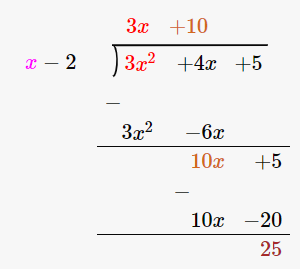∴ the Quotient is 3x + 10 and the Remainder is 25.

(ii) 10x2 – 7x + 8, 5x – 3

By using long division method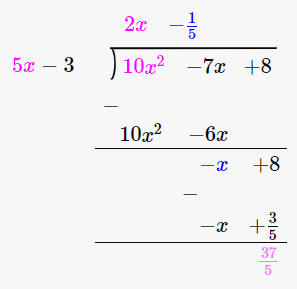∴ the Quotient is 2x – 1/5 and the Remainder is 37/5.

(iii) 5y3 – 6y2 + 6y – 1, 5y – 1

By using long division method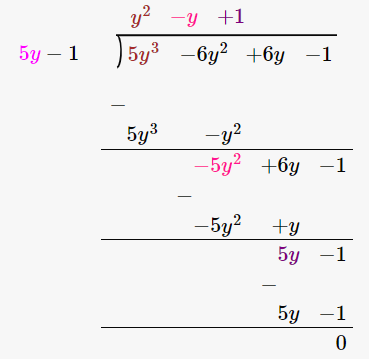∴ the Quotient is y2 – y + 1 and the Remainder is 0.

(iv) x4 – x3 + 5x, x – 1

By using long division method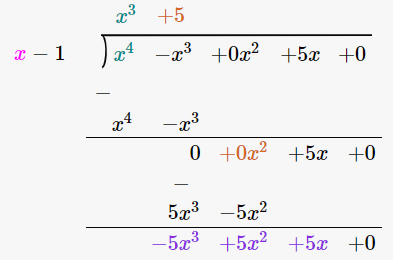∴ the Quotient is x3 + 5 and the Remainder is 5.

(v) y4 + y2, y2 – 2

By using long division method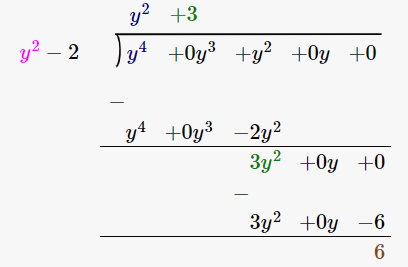∴ the Quotient is y2 + 3 and the Remainder is 6.

2. Find Whether or not the first polynomial is a factor of the second:

(i) x + 1, 2x2 + 5x + 4

(ii) y – 2, 3y3 + 5y2 + 5y + 2

(iii) 4x2 – 5, 4x4 + 7x2 + 15

(iv) 4 – z, 3z2 – 13z + 4

(v) 2a – 3, 10a2 – 9a – 5

(vi) 4y + 1, 8y2 – 2y + 1

Solution:

(i) x + 1, 2x2 + 5x + 4

Let us perform long division method,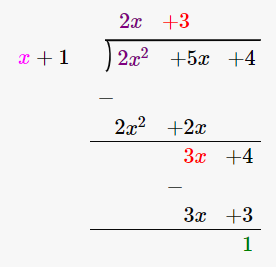Since remainder is 1 therefore the first polynomial is not a factor of the second polynomial.

(ii) y – 2, 3y3 + 5y2 + 5y + 2

Let us perform long division method,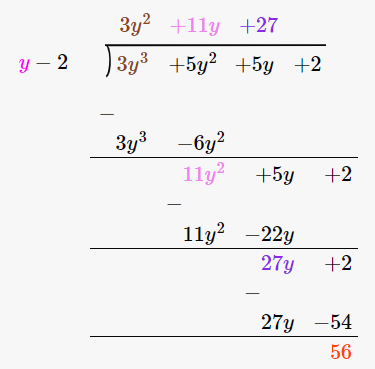Since remainder is 56 therefore the first polynomial is not a factor of the second polynomial.

(iii) 4x2 – 5, 4x4 + 7x2 + 15

Let us perform long division method,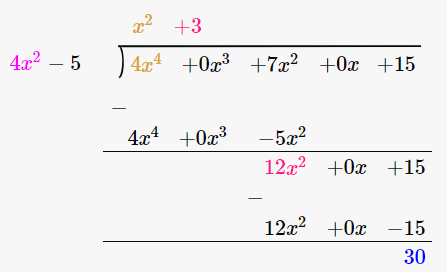Since remainder is 30 therefore the first polynomial is not a factor of the second polynomial.

(iv) 4 – z, 3z2 – 13z + 4

Let us perform long division method,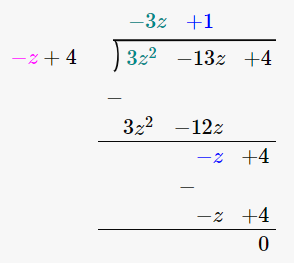Since remainder is 0 therefore the first polynomial is a factor of the second polynomial.

(v) 2a – 3, 10a2 – 9a – 5

Let us perform long division method,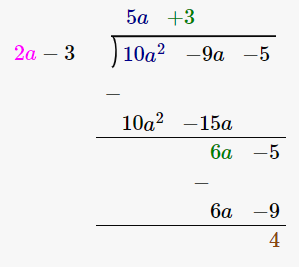Since remainder is 4 therefore the first polynomial is not a factor of the second polynomial.

(vi) 4y + 1, 8y2 – 2y + 1

Let us perform long division method,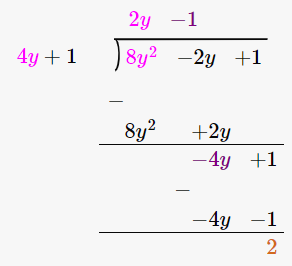Since remainder is 2 therefore the first polynomial is not a factor of the second polynomial.